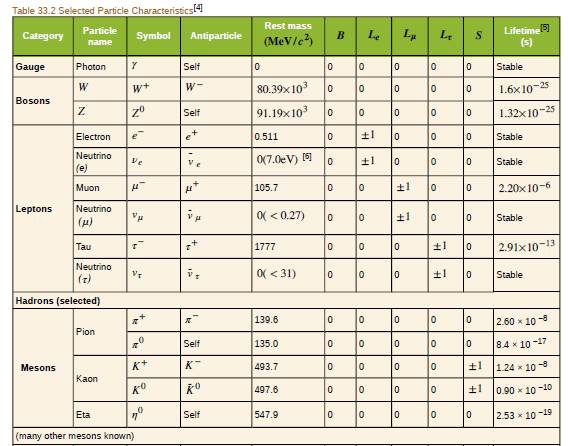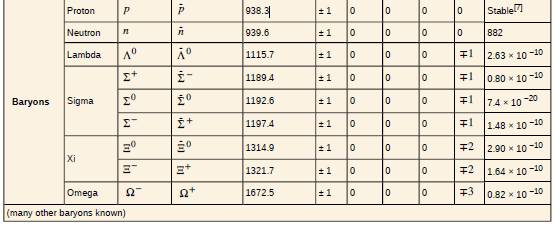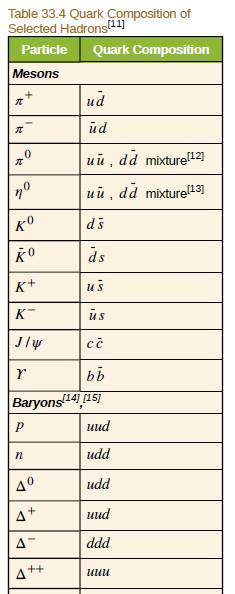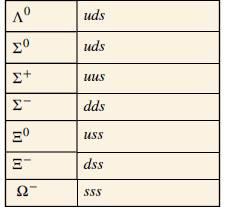Chapter 33, Problem 35PE

Chapter
Section
Textbook Problem

Verify the quantum numbers given for the Ω + in Table 33.2 by adding the quantum numbers for its quark constituents as inferred from Table 33.4.To determine

To verify the quantum numbers of Ω+ particle with the help of quark structure.

Explanation

Given info:

Quarks are fundamental particles and the quark structure of Ω+ particle is s¯s¯s¯.

Calculation:

For Ω+ particle. B=1 , S=+3 and charge =+e

We can verify this quantum numbers with the help of quarks.

Quark structure of Ω+ particle is s¯s¯s¯.

Baryon number of quark s¯=13

So, baryon number of Ω+ particle =13+1

Still sussing out bartleby?

Check out a sample textbook solution.

See a sample solution

The Solution to Your Study Problems

Bartleby provides explanations to thousands of textbook problems written by our experts, many with advanced degrees!

Get Started

The kidneysworking units are a. photons. b. genes. c. nephrons. d. villi.

Nutrition: Concepts and Controversies - Standalone book (MindTap Course List)

Give each of the following amines an IUPAC name: a. b. c.

Chemistry for Today: General, Organic, and Biochemistry

Describe four of the nutritional benefits of human milk to the infant.

Nutrition Through the Life Cycle (MindTap Course List)